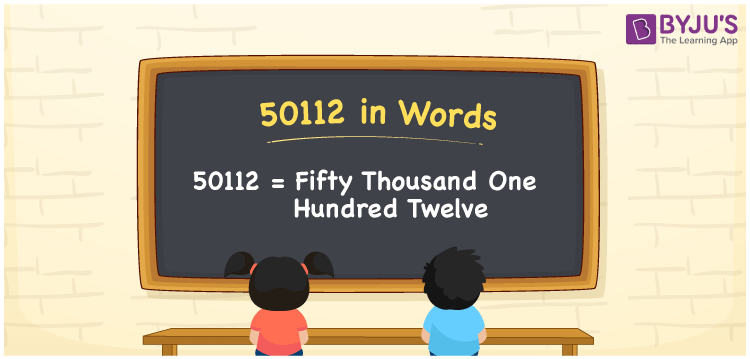# 50112 in Words

We can write 50112 in words is Fifty thousand one hundred twelve. If you donated Rs. 50112 to an NGO, you may communicate this as, “I donated Fifty thousand one hundred twelve rupees to an NGO”. As we know, we can easily derive the number 50112 into words using a place value chart. In this article, you will quickly understand how to write the cardinal number 50112 in word form.

 50112 in words Fifty thousand one hundred twelve Fifty thousand one hundred twelve in Numbers 50112

## 50112 in English words

We generally express numbers in words using the English alphabet. So, we can write 50112 in English as “Fifty thousand one hundred twelve”.## How to Write 50112 in Words?

The below table represents the place value chart for the number 50112. It has five columns to define the place values for all the five digits of the number 50112.

 Ten thousand Thousands Hundreds Tens Ones 5 0 1 1 2

Here, ones = 2, tens = 1, hundreds = 1, thousands = 0, ten thousands = 5.

These numbers can be expanded as given below.

5 × Ten thousand + 0 × Thousand + 1 × Hundred + 1 × Ten + 2 × One

= 5 × 10000 + 0 × 1000 + 1 × 100 + 1 × 10 + 2 × 1

= 50000 + 100 + 10 + 2

= 50000 + 100 + 12

= Fifty thousand + One hundred + Twelve

= Fifty thousand one hundred twelve

Therefore, 50112 in words is written as Fifty thousand one hundred twelve.

50112 is a natural number that is the successor of 50111 and the predecessor of 50113.

50112 in words – Fifty thousand one hundred twelve

Is 50112 an odd number? – No

Is 50112 an even number? – Yes

Is 50112 a perfect square number? – No

Is 50112 a perfect cube number? – No

Is 50112 a prime number? – No

Is 50112 a composite number? – Yes

## Frequently Asked Questions on 50112 in Words

### How do you write 50112 in words?

We can write the number 50112 in words as Fifty thousand one hundred twelve.

### How to write an amount of Rs. 50112 in words on a cheque?

On a cheque, we must write an amount of Rs. 50112 in words as “Fifty thousand one hundred twelve rupees only”.

### What number is Fifty thousand one hundred twelve in words?

The number 50112 is equivalent to Fifty thousand one hundred twelve in words, i.e. 50112 = Fifty thousand one hundred twelve.